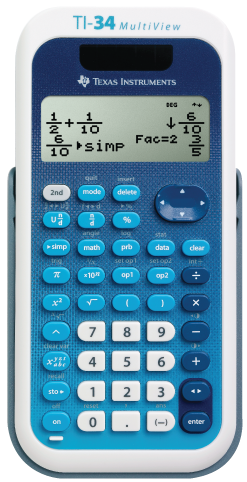Goldwise Development Limited# TI-34 MultiView

Key features

• Four-line display
• One- and two-variable statistics
• MathPrint™ feature
• Step-by-step fraction simplification
• Edit, cut, and paste entries
• Solar and battery powered

Exam acceptance

The TI-34 MultiView™ scientific calculator is approved for use on SAT*, ACT*, and AP* exams.

*SAT, ACT, and AP are registered trademarks of the College Board, which was not involved in the production of and does not endorse this product. Policies subject to change. Visit www.collegeboard.com

Ideal for

• Middle School math
• Pre-Algebra
• Algebra 1 and 2
• General science
• Geometry

Built-in functionality

• Review and edit previous entries via a scrollable home screen
• Paste inputs or outputs into new calculations
• MathPrint™ mode for input in math notation, including π, square roots, fractions, percents and exponents plus math notation output for fractions
• CLASSIC mode for similar entry and compatibility with previous two-line scientific models like the TI-34II pixels
• π symbol on input, some calculations in terms of pi and pi to the maximum decimal places of the calculator
• Toggle key to change the form of answers between fraction and decimal, or between π terms and decimal
• Fraction/decimal/percent conversions
• Change between improper fractions and mixed numbers, plus a mode setting for mixed number or improper fraction output as the default
• Simplification of fractions feature to simplify a fraction one factor at a time instead of automatically simplifying to lowest terms
• Integer division denotes quotient and remainder
• Random number and random integer generator
• MODE menu for selecting calculator mode settings
• Functions accessed directly through keys or through vertical menus
• Negation key
• Two constant operation features for exploring mathematical patterns
• Combinations and permutations
• Trigonometry functions including sin, cos, tan, inverse sin, inverse cos and inverse tan
• Logs and antilogs
• Convert angles from degrees to radians
• Square root function is primary key
• %, x², ¹/x, π, x!, exponent key
• Fixed decimal mode option
• Basic Data/List Editor with three lists
• One- and two-variable statistics with stat variable input storage
• EOS: (Equation Operating System)
• Up to eight pending operations
• Up to 23 levels of parentheses
• Error recovery capability
• Quick/easy reset of calculator via 2 key press or menu for exam purposes
• Seven memory variables. (x,y,z,t,a,b,c)
• Scientific notation
• x10n key for quick input of numbers in powers of 10